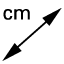# Constructing Tangents: Ex. 12

## DIRECTIONS:

In the applet below, note the circle with center A. You can alter the radius by dragging the orange point on the circle. Point B is a point on the circle. Point C is a point that lies outside the circle. Feel free to alter the locations of any of these 4 points at any time. 1) Use the TANGENTStool to construct a line through B that is tangent to the circle. 2) Use the TANGENTS tool to construct a line passing through C that is tangent to the circle. What do you notice? 3) Use the INTERSECTtool to plot the point(s) at which these tangents intersect the circle. GeoGebra should name these points D and E. Further directions appear below the applet.
4) Construct 2 radii with endpoint A (obviously) and other endpoint located at each of the points you constructed in step (3).

## 5)

Measure the angle at which each radius meets each tangent. What do you notice?

## 6)

Use the DISTANCE OR LENGTHtool to measure the distance from C to D and the distance from C to E. What do you notice?

When you're done (or if you're unsure of something) feel free to check by watching the brief silent screencast below the applet.